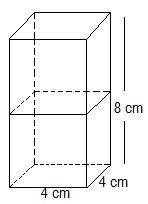Newbie

# 2 cubes each of volume 64 cm3 are joined end to end. Find the surface area of the resulting cuboid. Q.1

• 2

Today i am solving the ncert class 10 chapter surface areas and volumes its very tricky question to solve . Find the solution of exercise 13.1 question number 1 give me the simplest solution of this question also find the best solution of this question.2 cubes each of volume 64 cm3 are joined end to end. Find the surface area of the resulting cuboid.

Share

1. The diagram is given as:Given,

The Volume (V) of each cube is = 64 cm3

This implies that a3 = 64 cm3

∴ a = 4 cm

Now, the side of the cube = a = 4 cm

Also, the length and breadth of the resulting cuboid will be 4 cm each. While its height will be 8 cm.

So, the surface area of the cuboid = 2(lb+bh+lh)

= 2(8×4+4×4+4×8) cm2

= 2(32+16+32) cm2

= (2×80) cm2 = 160 cm2

• 0